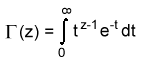The SDL Component Suite is an industry leading collection of components supporting scientific and engineering computing. Please visit the SDL Web site for more information....## LnGamma

 Unit: SDL_statis Class: none Declaration: function LnGamma (x: double): double;

The function LnGamma returns the natural logarithm of the Gamma function. The parameter x may assume any values greater than zero, for all other values the function returns a zero value. The Gamma function is defined as follows:Hint: If you are using the LnGamma function for calculating factorials you may apply the round function for small arguments in order to get exact values: fact(x) := round(exp(LnGamma(x)); However, it is more efficient to calculate the factorial by just multiplying the series of numbers as long as the argument is below 10.

Note: The Gamma function is calculated according to a formula given by Lanczos, which gives better numerical precision than by using a series expansion. Precision is about 8 decimal places (for arguments x > 1).

Last Update: 2012-Oct-20